Question

# 5. Draw the magnitude characteristic of the Bode plot of the following transfer function: H(s)10(10s +11...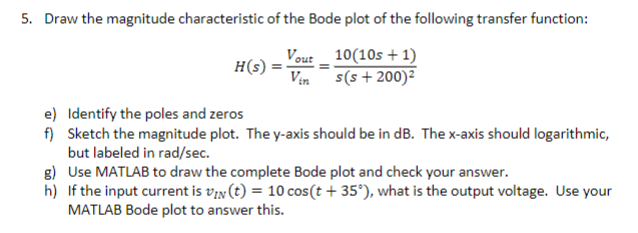5. Draw the magnitude characteristic of the Bode plot of the following transfer function: H(s)10(10s +11 (S + 200)2 Identify the poles and zeros Sketch the magnitude plot. The y-axis should be in dB. The x-axis should logarithmic, but labeled in rad/sec e) f) g) Use MATLAB to draw the complete Bode plot and check your answer. h) If the input current is VIN(t)-10 cos(t+35"),what is the output voltage. Use your MATLAB Bode plot to answer this.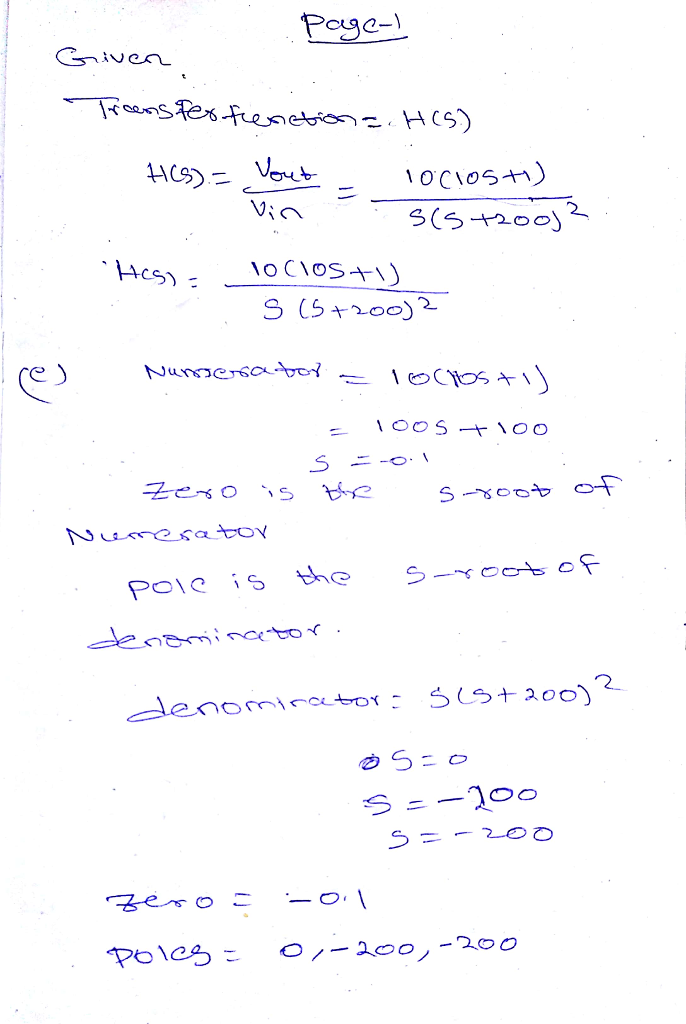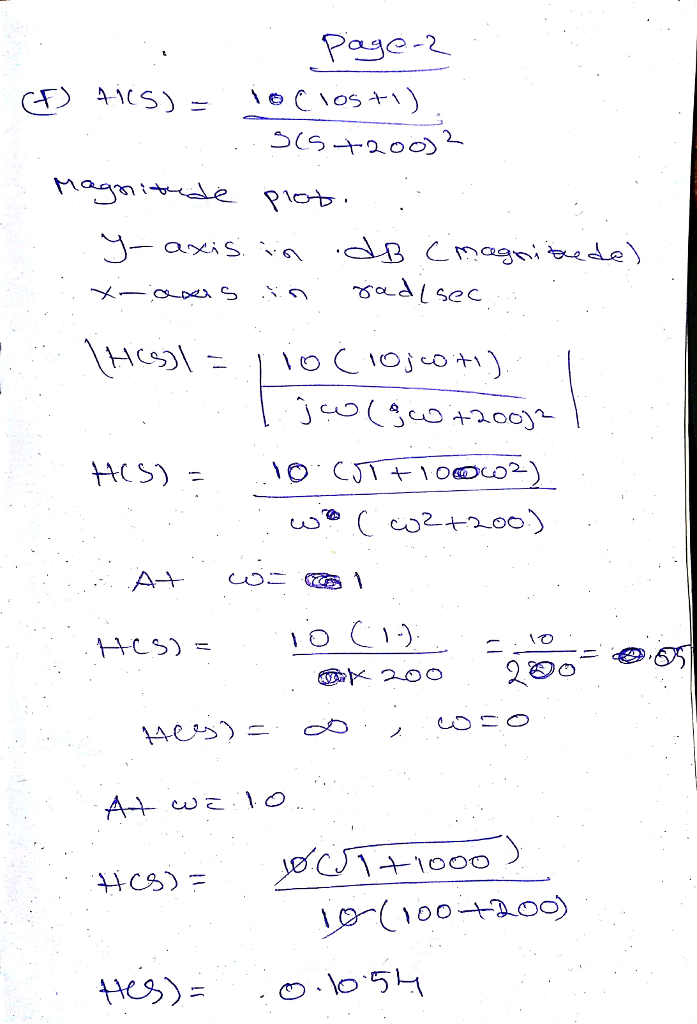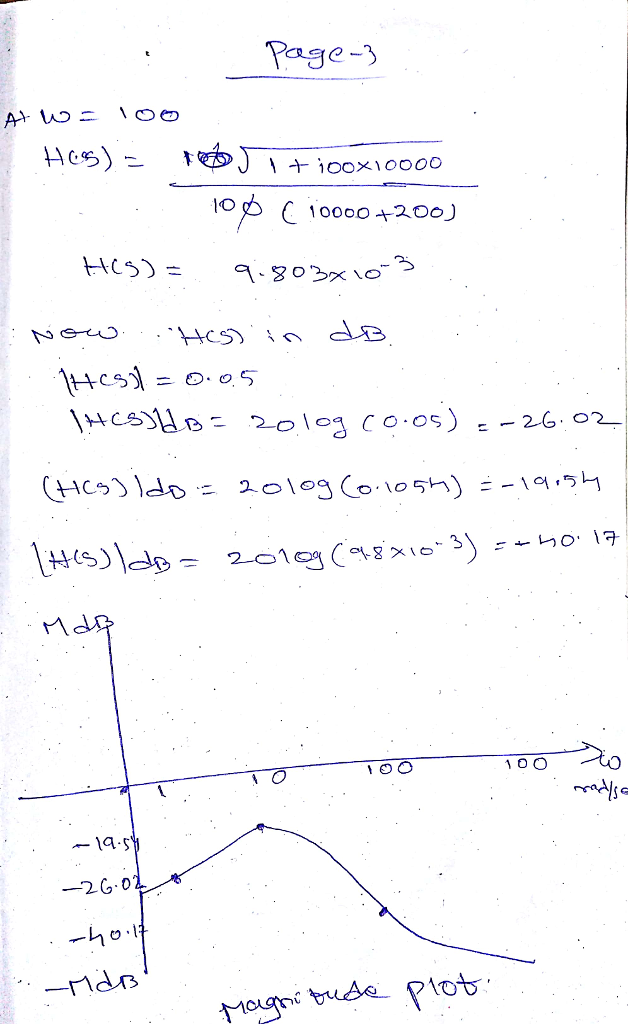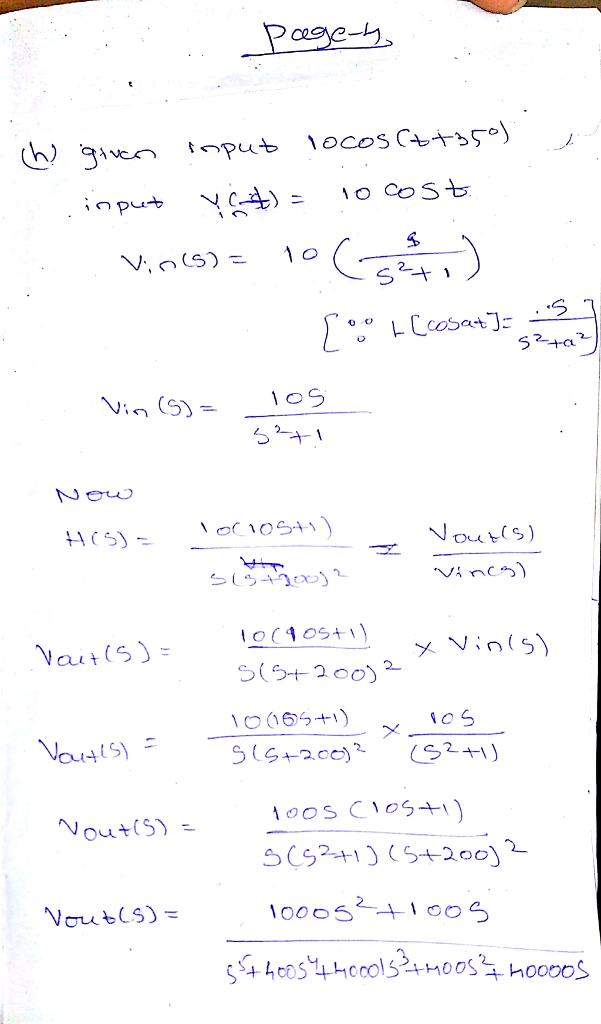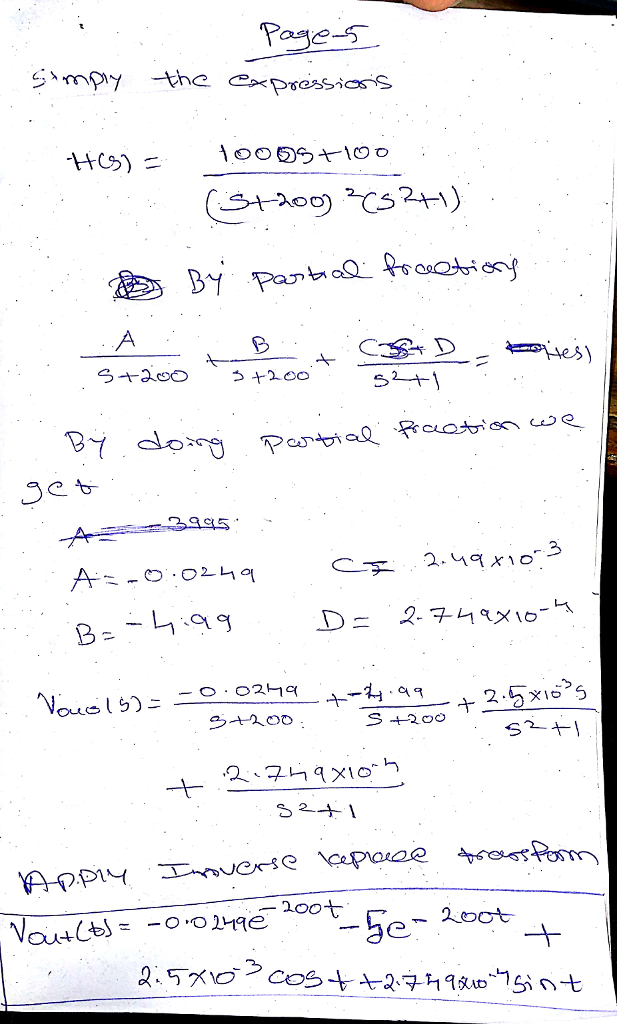#### Earn Coins

Coins can be redeemed for fabulous gifts.

Similar Homework Help Questions
• ### 4. Draw the magnitude characteristic of the Bode plot of the following transfer function: 10s H...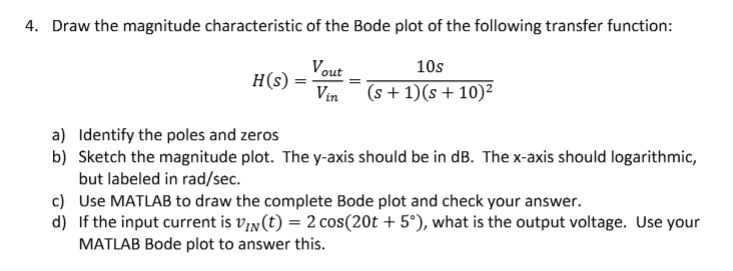4. Draw the magnitude characteristic of the Bode plot of the following transfer function: 10s H (s) =-= (s+1)(s+102 L7n Identify the poles and zeros Sketch the magnitude plot. The y-axis should be in dB. The x-axis should logarithmic, but labeled in rad/sec Use MATLAB to draw the complete Bode plot and check your answer. If the input current is un(t) = 2 cos(20t + 5°), what is the output voltage. Use your MATLAB Bode plot to answer this a)...

• ### please answer all questions and show all steps 3. Draw the magnitude characteristic of the Bode...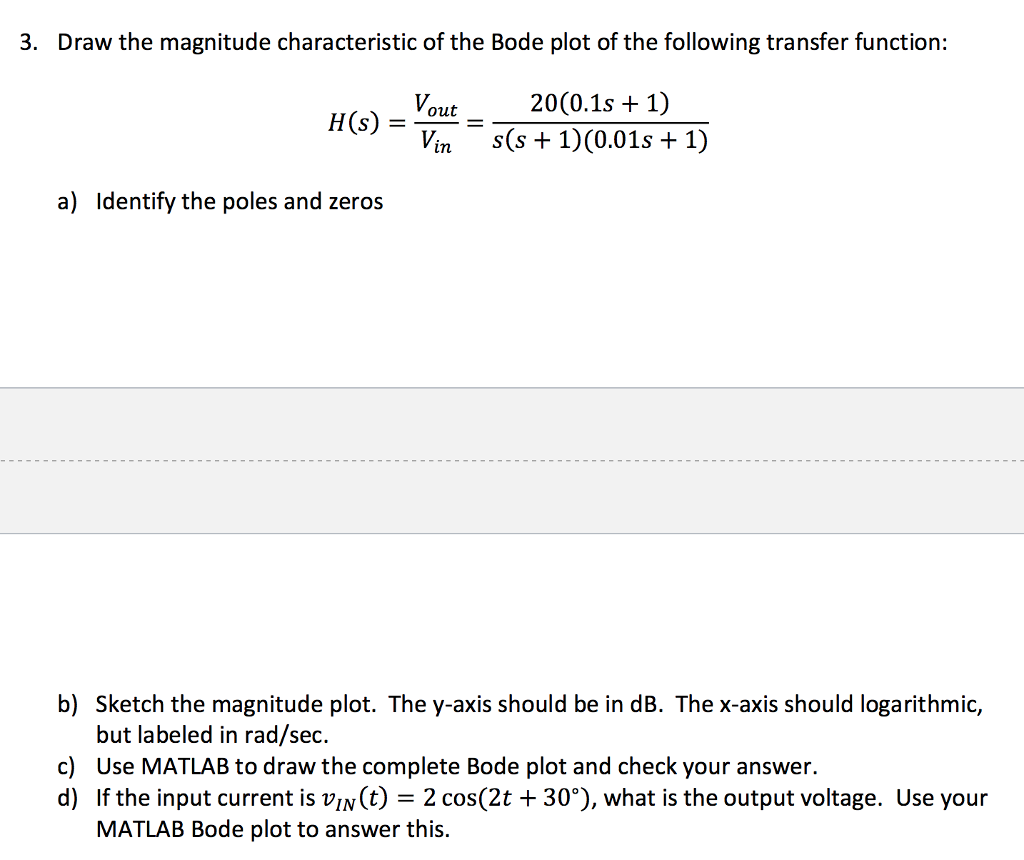please answer all questions and show all steps 3. Draw the magnitude characteristic of the Bode plot of the following transfer function: Hsout20(0.1s + 1 Vin S(s1)(0.01s +1) a) ldentify the poles and zeros b) Sketch the magnitude plot. The y-axis should be in dB. The x-axis should logarithmic, but labeled in rad/sec. Use MATLAB to draw the complete Bode plot and check your answer. If the input current is uln(t)-2 cos(2t + 30%), what is the output voltage. Use...

• ### Hw 10 Problem 2 2. Draw the magnitude characteristic of the Bode plot of the following...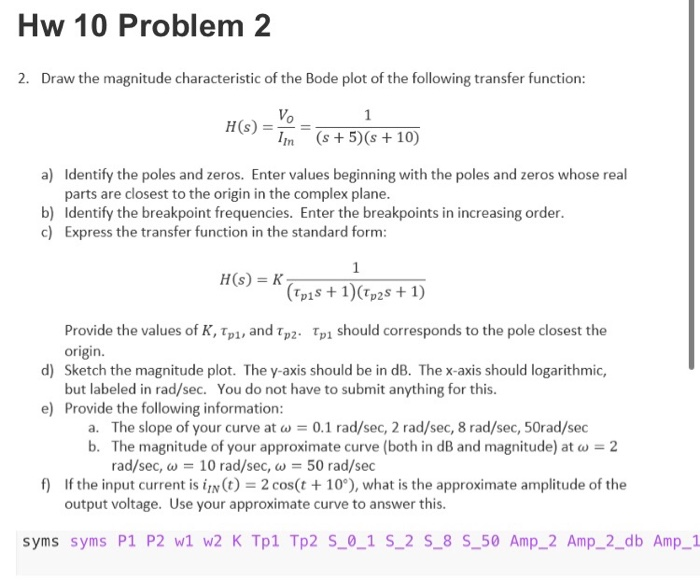Hw 10 Problem 2 2. Draw the magnitude characteristic of the Bode plot of the following transfer function: HG) = (s +5)(s +10) 5 + 5 + 10) a) Identify the poles and zeros. Enter values beginning with the poles and zeros whose real parts are closest to the origin in the complex plane. b) Identify the breakpoint frequencies. Enter the breakpoints in increasing order. c) Express the transfer function in the standard form: H(S) = (Tpis + 1)(Tp25 +...

• ### Chapter 12, Problem 12.21 (Multistep) Part 1 Correct. Sketch the magnitude characteristic of the Bode plot...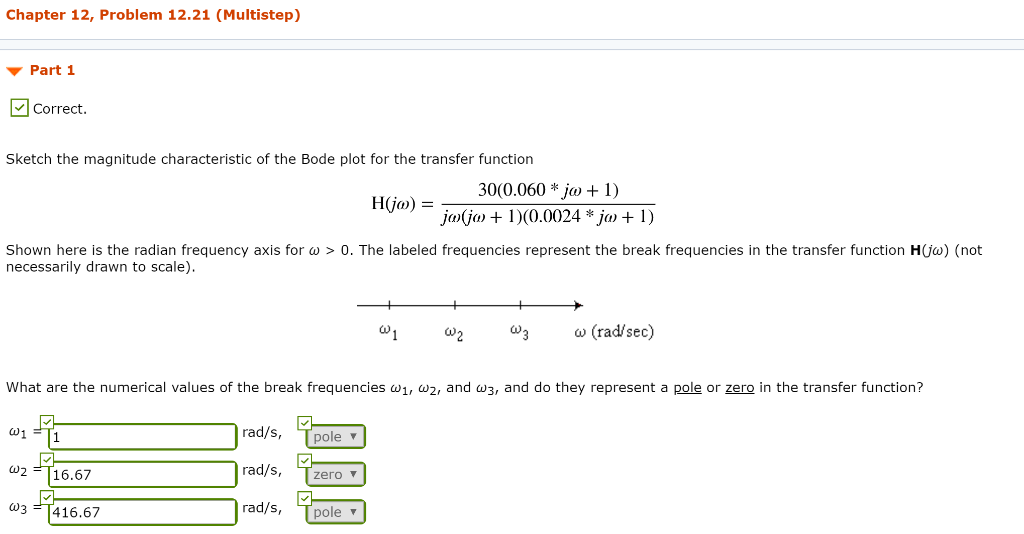Chapter 12, Problem 12.21 (Multistep) Part 1 Correct. Sketch the magnitude characteristic of the Bode plot for the transfer function 30(0.060 * jo + 1) jojo + 1)(0.0024 * ja + 1) H0) = Shown here is the radian frequency axis for w > 0. The labeled frequencies represent the break frequencies in the transfer function H(o) (not necessarily drawn to scale). -+ W + w W3 W (rad/sec) What are the numerical values of the break frequencies w1, wz,...

• ### 4. Sketch the Bode magnitude plot ofa fiter having a transfer function 10's H(s)- (s+500) (s+S0s+...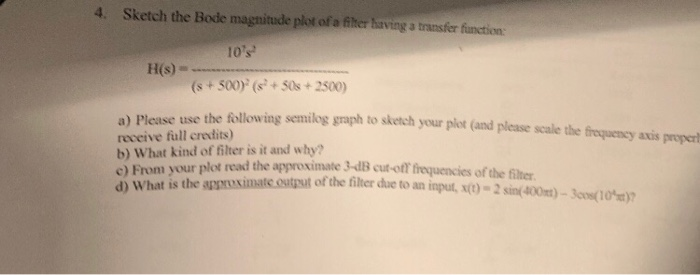4. Sketch the Bode magnitude plot ofa fiter having a transfer function 10's H(s)- (s+500) (s+S0s+2500) he following senilog graph to sketch yo ur plot (and please scale the eequeney axis proper a) Please use t receive full credits) b) What kind of filter is it and why? read the approximate 3-dB cut-off frequencies of the filter From your plot ont is the asrsximate.ostt of the filter due to an input, st)- 2sin(400)-3os(10 a) c) frequency axis properly a) Please...

• ### Sketch the bode plot of a signal conditioner with the transfer function G(s) in the provided...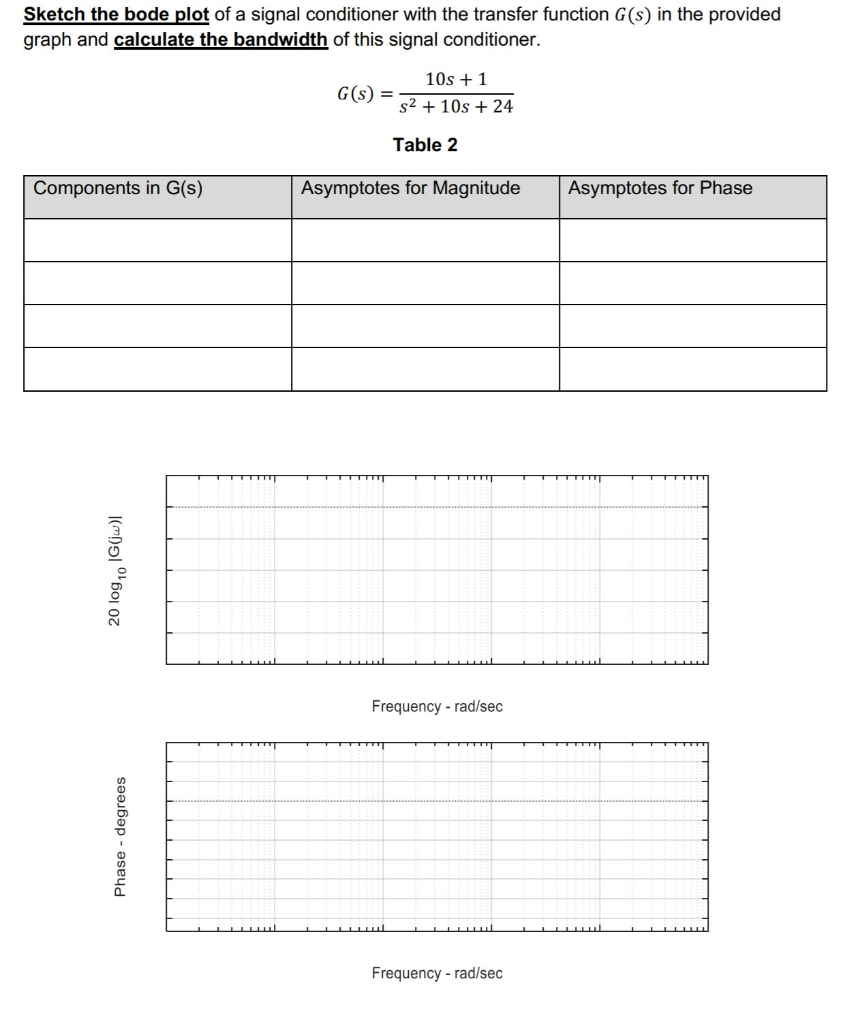Sketch the bode plot of a signal conditioner with the transfer function G(s) in the provided graph and calculate the bandwidth of this signal conditioner. GO 10s +1 S2 + 10s + 24 Table 2 Components in G(S) Asymptotes for Magnitude Asymptotes for Phase 20 log,0 1G(jw) Frequency-rad/sec Phase - degrees Frequency - rad/sec

• ### Chapter 12, Problem 12.16 Describe the sketch of the magnitude characteristic of the Bode plot for...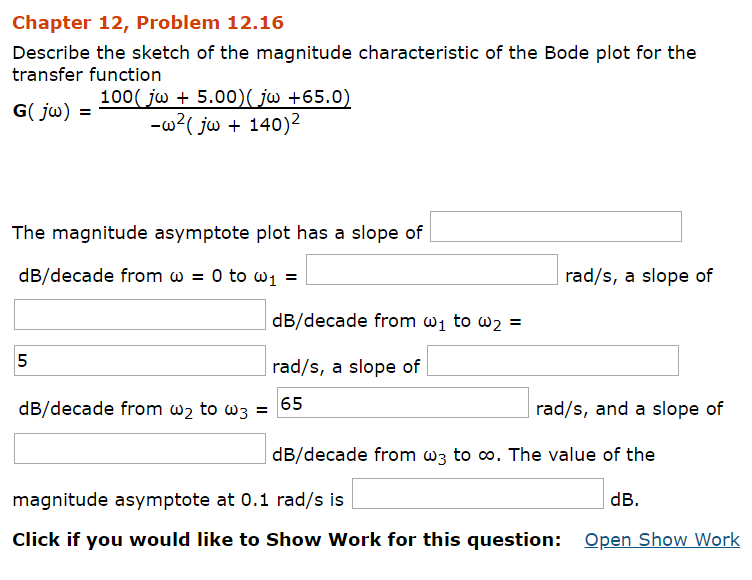Chapter 12, Problem 12.16 Describe the sketch of the magnitude characteristic of the Bode plot for the transfer function The magnitude asymptote plot has a slope of dB/decade from ω-0 to ω! rad/s, a slope of dB/decade from ω1 to ω2- 5 rad/s, a slope of dB/decade from ω2 to ω3-65 rad/s, and a slope of dB/decade from w3 to oo. The value of the magnitude asymptote at 0.1 rad/s is dB. Click if you would like to Show Work...

• ### QUESTION #2 PLEASE 1. Derive the transfer function for the circuit shown below. Plot H(s) versus...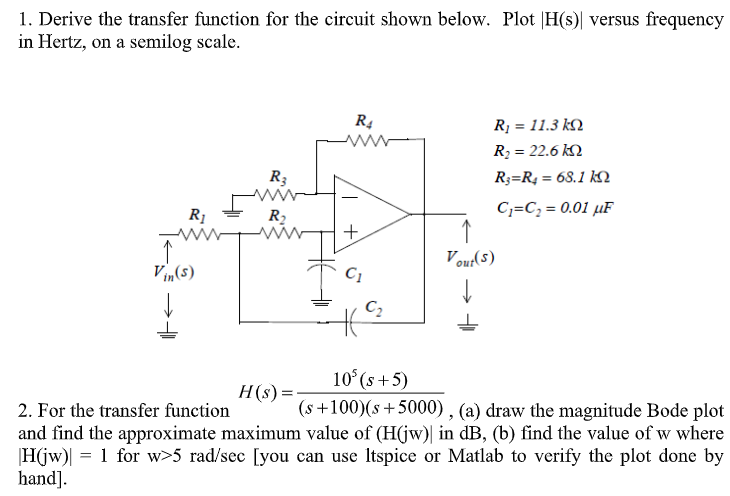QUESTION #2 PLEASE 1. Derive the transfer function for the circuit shown below. Plot H(s) versus frequency in Hertz, on a semilog scale. Ri 11.3 k Ri 22.6 k R R = 68.1 kN R3 C C 0.01 uF R2 Vout(s) Vin(s) C2 10 (s+5) H(s) = (s+100)(s5000) , (a) draw the magnitude Bode plot 2. For the transfer function and find the approximate maximum value of (H(jw) in dB, (b) find the value of w where 1 for w>5...

• ### 3. Draw the Bode plot (magnitude and phase) for the transfer function H(s) of a CT...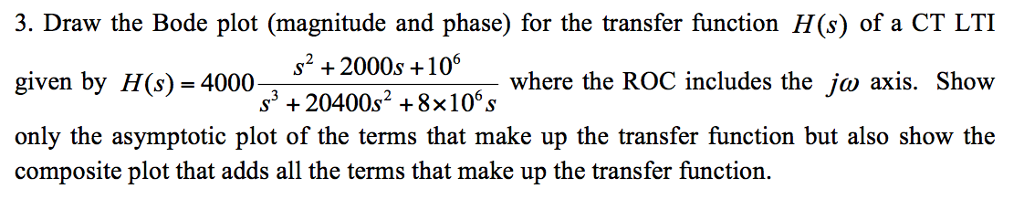3. Draw the Bode plot (magnitude and phase) for the transfer function H(s) of a CT LTI given by H(s) 4000 only the asymptotic plot of the terms that make up the transfer function but also show the composite plot that adds all the terms that make up the transfer function. S+2000s+10 where the ROC includes the ja axis. Show

• ### -... Find the transfer function H(w) with the Bode magnitude plot shown in Fig. 14.74 H...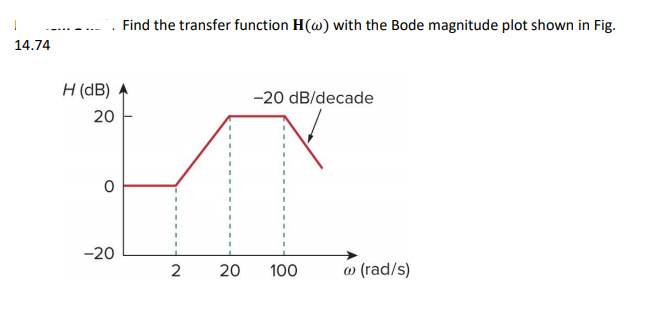-... Find the transfer function H(w) with the Bode magnitude plot shown in Fig. 14.74 H (dB)参 20 20 dB/decade 0 -20L 2 20 100 a) (rad/s)